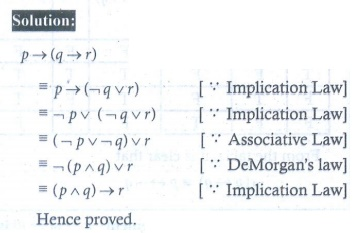Home | | Maths 12th Std | Exercise 12.2: Mathematical Logic

# Exercise 12.2: Mathematical Logic

Maths Book back answers and solution for Exercise questions - Mathematics : Discrete Mathematics: Mathematical Logic: Exercise Problem Questions with Answer, Solution

EXERCISE 12.2

1. Let p : Jupiter is a planet and q : India is an island be any two simple statements. Give  verbal sentence describing each of the following statements.

(i) ¬p (ii) p  ¬q  (iii) ¬p  q (iv) p → ¬q (v) p  q2. Write each of the following sentences in symbolic form using statement variables p and q .

(i) 19 is not a prime number and all the angles of a triangle are equal.

(ii) 19 is a prime number or all the angles of a triangle are not equal

(iii) 19 is a prime number and all the angles of a triangle are equal

(iv) 19 is not a prime number3. Determine the truth value of each of the following statements

(i) If 6 + 2 = 5 , then the milk is white.

(ii) China is in Europe or √3 is an integer

(iii) It is not true that 5 + 5 = 9 or Earth is a planet

(iv) 11 is a prime number and all the sides of a rectangle are equal4. Which one of the following sentences is a proposition?

(i) 4 + 7 = 12 (ii) What are you doing? (iii) 3n  81, n ∈ ℕ (iv) Peacock is our national bird (v) How tall this mountain is!5. Write the converse, inverse, and contrapositive of each of the following implication.

(i) If x and y are numbers such that x = y , then x2 = y2

(ii) If a quadrilateral is a square then it is a rectangle6. Construct the truth table for the following statements.

(i) ¬ p ∧ ¬q (ii) ¬p ∧ ¬q) (iii) ( p  q ) ¬q (iv) (¬ p  r ( p  q)7. Verify whether the following compound propositions are tautologies or contradictions or Contingency

(i) ( p  q ) ¬p  q)

(ii) ( ( p  q¬p )  q

(iii) ( p  q )  (¬ p  q)

(iv) ( ( p  q )  (q  r))→ ( p  r)8. Show that (i) ¬p  q≡ ¬p ¬q  (ii) ¬p  q p ¬q .9. Prove that q  p ≡¬ p →¬q10. Show that p  q and q  p are not equivalent11. Show that ¬p  q p ↔¬q12. Check whether the statement p  ( q  p) is a tautology or a contradiction without using the truth table.13. Using truth table check whether the statements¬p  q )  (¬p  q) and ¬p are logically equivalent.14. Prove p  ( q  r) ≡ ( p  q r without using truth table.15. Prove that p  (¬q  r ) ≡ ¬p  (¬q ∨ r) using truth table.1. (i) ¬p : Jupiter is not a planet (ii) p  ¬q : Jupiter is a planet and India is not an Island.

(iii) ¬ p  q : Jupiter is not a planet or India is an Island.

(iv) p → ¬q : If Jupiter is a planet then India is not an Island.

(v) p ↔ q Jupiter is a planet if and only if India is an Island.

2. (i) ¬ p  q     (ii) p ∨ ¬q   (iii) p  q  (iv) ¬p

3. (i) p → q is T (ii)   is F (iii) ¬  q is T (iv) p  q  is F

4. (i), (iii) and (iv) are propositions

5. (i) Converse: If x and y are numbers such that x2 = y2 then x = y .

Inverse: If x and y are numbers such that x  y then x2 ≠ y2.

Contra positive: If x and y are numbers such that x2 ≠ y2 then x  y .

(ii) Converse: If a quadrilateral is a rectangle then it is a square.

Inverse: If a quadrilateral is not a square then it is not a rectangle.

Contrapositive: If a quadrilateral is not a rectangle then it is not a square.

6.7. (i) Contradiction  (ii) Tautology  (iii) Contingency  (iv) Tautology

12. p → ( q → p) is a Tautology.

13. Yes. The statements are logically equivalent.

Tags : Problem Questions with Answer, Solution , 12th Maths : UNIT 12 : Discrete Mathematics
Study Material, Lecturing Notes, Assignment, Reference, Wiki description explanation, brief detail
12th Maths : UNIT 12 : Discrete Mathematics : Exercise 12.2: Mathematical Logic | Problem Questions with Answer, Solution